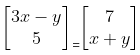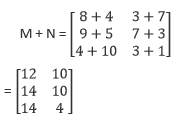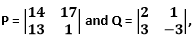# Frank Solutions for Chapter 11 Matrices Class 10 ICSE Mathematics

1. Classify the following matrices:

(v)The order of the given matrix is, 3 × 2.

Therefore, the given matrix is a rectangular matrix of 3 × 2.

The order of the given matrix is, 1 × 2.

Therefore, the given matrix is a rectangular matrix of 1 × 2.

The order of the given matrix is, 2 × 3.

Therefore, the given matrix is a rectangular matrix of 2 × 3.

The order of the given matrix is, 2 × 2.

Therefore, the given matrix is a square matrix of 2 × 2.

The order of the given matrix is, 3 × 4.

Therefore, the given matrix is a rectangular matrix of 3 × 4.

2. Find the values of a and b, if [2a + 3b a – b] = [19 2]

From the question,

2a + 3b = 19 …[equation (i)]

a – b = 2 …[equation (ii)]

⇒ a = 2 + b

Now, substitute the value of a in equation (i),

2(2 + b) + 3b = 19

⇒ 4 + 2b + 3b = 19

⇒ 5b = 19 – 4

⇒ 5b = 15

⇒ b = 15/5

⇒ b = 3

Then, a = 2 + b

= 2 + 3

= 5

Therefore, the value of a is 5 and b is 3.

Consider the given two matrices,

=The given two matrices are rectangular matrices of 2 × 1.

3x – y = 7 …[equation (i)]

x + y = 5 …[equation (ii)]

⇒ x = 5 – y

Now, substitute the value of x in equation (i),

3(5 – y) – y = 7

⇒ 15 – 3y – y = 7

⇒ 15 – 4y = 7

⇒ 15 – 7 = 4y

⇒ 8 = 4y

⇒ y = 8/4

⇒ y = 2

Then,

x + y = 5

⇒ x = 5 – y

⇒ x = 5 – 2

⇒ x = 3

Therefore, the value of x is 3 and y is 2.

From the given matrices,

a + 3b = 5 …[equation (i)]

2a – b = 3 …[equation (ii)]

⇒ b = 2a – 3

Now, substitute the value of b in equation (i), we get

a + 3(2a – 3) = 5

⇒ a + 6a – 9 = 5

By transposing,

7a = 5 + 9

⇒ 7a = 14

⇒ a = 14/7

⇒ a = 2

Again substitute the value of a in equation (i),

2 + 3b = 5

⇒ 3b = 5 – 2

⇒ 3b = 3

⇒ b = 3/3

⇒ b = 1

Then,

3c + d = 8 … [equation (iii)]

⇒ c – 2d = 5 … [equation (iv)]

⇒ c = 5 + 2d

Now substitute the value of c in equation (iii),

3(5 + 2d) + d = 8

⇒ 15 + 6d + d = 8

⇒ 7d = 8 – 15

⇒ 7d = – 7

d = -7/7

⇒ d = -1

substitute the value of d in equation (iv),

c – 2d = 5

⇒ c = 5 + 2d

⇒ c = 5 + 2(-1)

⇒ c = 5 – 2

⇒ c = 3

(i) A + B

(ii) 2A + 3B

(iii) A – 2B.

Given two matrices are square matrices of 2 × 2

6. If A = [4 7] and B = [3 1], find:

(i) A + 2B

(ii) A –B

(iii) 2A – 3B

From the question it is given that,

A = [4 7]1× 2

B = [3 1]1× 2

Then,

(i) A + 2B

2B = [3 × 2 1 × 2]

= [6 2]

So, A + 2B = [4 + 6 7 + 2]

= [10 9] 1× 2

(ii) A – B

A – B = [4 – 3 7 + 1]

= [1 6] 1× 2

(iii) 2A – 3B

2A = [4 × 2 7 × 2]

= [8 14]

3B = [3 × 3 1 × 3]

= [9 3]

So, 2A – 3B = [8 – 9 14 – 3]

= [-1 11] 1× 2

7.If P = and Q = find : (i) 2P + 3Q

(ii) 2Q – P

(iii) 3P – 2Q.

Given two matrices are square matrices of 2 × 2

Then,

(i) 2P + 3Q

Given two matrices are rectangular matrices of 2 × 3

Then,

(i) M + N
(ii) M – N

Given two matrices are rectangular matrices of 3 × 2

Then,

(i)(ii)

(i) negative A

(ii) A'

Given matrix is a rectangular matrix of 2 × 3.

Then,

Transpose of a matrix by switching its rows with its columns

(i) P’

(ii) P + P’

(iii) P – P’

(i)

12. If, find the transpose of matrix B and if possible find the sum of the two matrices. If not possible state the reason.

From the question it is given that,

We cannot add them, because to add two matrices their corresponding number of rows and number of columns should be same. But in the above case B and Bt are not same.

From the question it is given that,

Then,

5 + q = 9

⇒ q = 9 – 5

⇒ q = 4

r + 4 = 7

⇒ r = 7 – 4

⇒ r = 3

p + 3 = 5

⇒ p = 5 – 3

⇒ p = 2

7 + s = 8

⇒ s = 8 – 7

⇒ s = 1

From the question it is given that,

Then,

4p = 12

⇒ p = 12/4

⇒ p = 3

6q = 6

⇒ q = 6/6

⇒ q = 1

8 + 2q = 2r

⇒ 8 + 2(1) = 2r

⇒ 8 + 2 = 2r

⇒ r = 10/2

⇒ r = 5

12 = 3s

⇒ s = 12/3

⇒ s = 4

From the question it is given that,

Then,

2a + b = 4 …[equation (i)]

3a – b = 6 …[equation (ii)]

Now we have to add both equation (i) and equation (ii) we get,

5a = 10

⇒ a = 10/5

⇒ a = 2

substitute the value of a in equation (i) we get,

2(2) + b = 4

⇒ 4 + b = 4

⇒ b = 0

Then,

c = 3a

⇒ c = 3(2)

⇒ c = 6

⇒ d = 7

3a + 2b = 12 ...(1)

2a – b = 1 …(2)

⇒ b = 2a – 1

4p – 3q = 16 ...(3)

2p + q = 8 …(4)

Putting the value of b in (1)

3a + 4a – 2 = 12

⇒ 7a = 14

⇒ a = 2

from (2)

4 – b = 1

⇒ b = 3

putting the value of q in (3)

4p – 24 + 6p = 16

⇒ 10p = 40

⇒ p = 4

From (4)

8 + q = 8

⇒ q = 0

(i) A + X = B

(ii) 2A – X = B

18. Iffind matrix M such that P – M = 3Q.

### Exercise 11.2

1. Evaluate the following:

(iii)